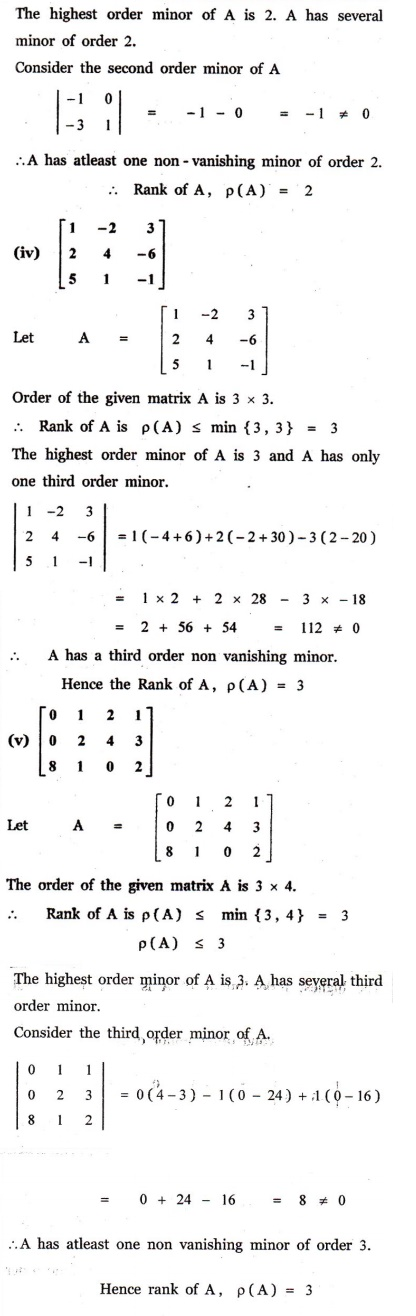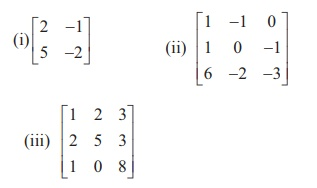Home | | Maths 12th Std | Exercise 1.2: Elementary Transformations of a Matrix

# Exercise 1.2: Elementary Transformations of a Matrix

Maths Book back answers and solution for Exercise questions - rank of thematrices by minor method, rank of the matrices by row reduction method, inverse of matrices by Gauss-Jordan method

EXERCISE 1.2

1. Find the rank of the following matrices by minor method:2. Find the rank of the following matrices by row reduction method:3. Find the inverse of each of the following by Gauss-Jordan method:1. (i) 1 (ii) 2 (iii) 2 (iv) 3 (v) 3

2. (i) 2 (ii) 3 (iii) 3Tags : Problem Questions with Answer, Solution , 12th Mathematics : UNIT 1 : Applications of Matrices and Determinants
Study Material, Lecturing Notes, Assignment, Reference, Wiki description explanation, brief detail
12th Mathematics : UNIT 1 : Applications of Matrices and Determinants : Exercise 1.2: Elementary Transformations of a Matrix | Problem Questions with Answer, Solution